﻿ 有理Bézier曲线及其在粗细不同管道拼接中的应用 Rational Bézier Curve and Its Application in Blending of Thickness Different Tubes

Vol. 07  No. 09 ( 2018 ), Article ID: 26703 , 6 pages
10.12677/AAM.2018.79130

Rational Bézier Curve and Its Application in Blending of Thickness Different Tubes

Taotuge1, Wurengaowa1, Fang Wang2, Genzhu Bai3*

1Inner Mongolia Tongliao Vocational College, Tongliao Inner Mongolia

2Zhejiang Changzheng Vocational & Technical College, Hangzhou Zhejiang

3Inner Mongolia University for the Nationalities, Tongliao Inner Mongolia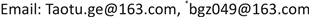Received: Aug. 13th, 2018; accepted: Aug. 29th, 2018; published: Sep. 5th, 2018ABSTRACT

In this paper, the rational Bézier curve can be adjusted and controlled in the shape of a curve near a particular control point. Then, on the basis of the smooth blending circular tubes with the two different radiuses, the axes of the tubes are non-coplanar lines. The blending tubes that meet the conditions of G0 and G1 are constructed separately. A new tubes blending is obtained. It has theoretical significance and application value.

Keywords:Shape Parameters, Rational Bézier Curve, Axis, Tubes, Smooth Blending

1内蒙古通辽职业学院，内蒙古 通辽

2浙江长征职业技术学院，浙江 杭州

3内蒙古民族大学，内蒙古 通辽1. 引言

${\Phi }_{1}:\left\{\begin{array}{l}x={X}_{1}+a\mathrm{cos}\phi +a\mathrm{sin}\phi ,\\ y={Y}_{1}+{B}_{1}s+a\mathrm{cos}\phi +a\mathrm{sin}\phi ,\\ z=a\mathrm{cos}\phi +a\mathrm{sin}\phi .\end{array}$ (1)

${L}_{1}:\left\{\begin{array}{l}x={X}_{1}+0\cdot s,\\ y={Y}_{1}+{B}_{1}s,\\ z=0+0\cdot s,\end{array}$${L}_{2}:\left\{\begin{array}{l}x=0+0\cdot s,\\ y={Y}_{2}+0\cdot s,\\ z={Z}_{2}+{C}_{2}s.\end{array}$ (2)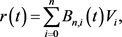$r\left(t\right)=\frac{\underset{i=0}{\overset{n}{\sum }}{B}_{n,i}\left(t\right){w}_{i}{V}_{i}}{\underset{i=0}{\overset{n}{\sum }}{B}_{n,i}\left(t\right){w}_{i}}$

n为次Bézier曲线和n次有理Bézier曲线。其中 ${B}_{n,i}\left(t\right),i=0,1,\cdots ,n$ 为Bernstein基函数。 ${w}_{i}\ne 0,i=0,1,\cdots ,n$ 为对应控制顶点的权因子。有理Bézier曲线和Bézier曲线一样通过首、末顶点并和特征多边形的首、末两条边相切。(3)

$\Phi :\left\{\begin{array}{l}x=\underset{i=0}{\overset{3}{\sum }}{B}_{3,i}\left(s\right){x}_{i}+r{N}_{1}\left(s\right)\mathrm{cos}\phi +r{B}_{1}\left(s\right)\mathrm{sin}\phi ,\\ y=\underset{i=0}{\overset{3}{\sum }}{B}_{3,i}\left(s\right){y}_{i}+r{N}_{2}\left(s\right)\mathrm{cos}\phi +r{B}_{2}\left(s\right)\mathrm{sin}\phi ,\\ z=\underset{i=0}{\overset{3}{\sum }}{B}_{3,i}\left(s\right){z}_{i}+r{N}_{3}\left(s\right)\mathrm{cos}\phi +r{B}_{3}\left(s\right)\mathrm{sin}\phi .\end{array}\text{}s\in \left[0,1\right],\text{}\phi \in \left(0,\text{π}\right).$ (4)

$p\left(s,\phi \right)=\left\{\begin{array}{l}x\left(s\right)+a{N}_{1}\left(s\right)\mathrm{cos}\phi +a{B}_{1}\left(s\right)\mathrm{sin}\phi ,\\ y\left(s\right)+a{N}_{2}\left(s\right)\mathrm{cos}\phi +a{B}_{2}\left(s\right)\mathrm{sin}\phi ,s\in \left[0,1\right],\text{}\phi \in \left(0,\text{π}\right)\\ z\left(s\right)+a{N}_{3}\left(s\right)\mathrm{cos}\phi +a{B}_{3}\left(s\right)\mathrm{sin}\phi .\end{array}$

2. 构造粗细不同的轴线异面管道的G0-拼接管道

1) 存在光滑拼接两个管道的轴线 ${\gamma }_{1}\left(s\right)$${\gamma }_{2}\left(s\right)$ 的有理Bézier曲线 $\gamma \left(s\right)$ ，即

${\gamma }_{1}\left(1\right)=\gamma \left(0\right),\gamma \left(1\right)={\gamma }_{2}\left(0\right).$

2) 在轴线光滑拼接点与轴线垂直的平面处的管道半径相同，即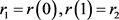$p\left(s,\phi \right)=\left\{\begin{array}{l}x\left(s\right)+\alpha \left(s\right){N}_{1}\left(s\right)\mathrm{cos}\phi +\alpha \left(s\right){B}_{1}\left(s\right)\mathrm{sin}\phi ,\\ y\left(s\right)+\alpha \left(s\right){N}_{2}\left(s\right)\mathrm{cos}\phi +\alpha \left(s\right){B}_{2}\left(s\right)\mathrm{sin}\phi ,s\in \left[0,1\right],\text{}\phi \in \left(0,\text{π}\right).\\ z\left(s\right)+\alpha \left(s\right){N}_{3}\left(s\right)\mathrm{cos}\phi +\alpha \left(s\right){B}_{3}\left(s\right)\mathrm{sin}\phi .\end{array}$

3. 构造光滑拼接粗细不同的轴线异面管道的G1-拼接管道

1) 存在光滑拼接两个轴线异面管道的轴线 ${\gamma }_{1}\left(u\right)$${\gamma }_{2}\left(u\right)$ 的有理Bézier曲线 $\gamma \left(u\right)$ ，使得 ${\gamma }_{1}\left(1\right)=\gamma \left(0\right),\gamma \left(1\right)={\gamma }_{2}\left(0\right).$

2) 存在光滑拼接两个轴线异面管道的母线 ${{\gamma }^{\prime }}_{1}\left(u\right)$${{\gamma }^{\prime }}_{2}\left(u\right)$ 的有理Bézier曲线 ${\gamma }^{\prime }\left(u\right)$ ，使得 ${{\gamma }^{\prime }}_{1}\left(1\right)={\gamma }^{\prime }\left(0\right),{\gamma }^{\prime }\left(1\right)={{\gamma }^{\prime }}_{2}\left(0\right)$

$p\left(s,\phi \right)=\left\{\begin{array}{l}x\left(s\right)+d\left(s\right){N}_{1}\left(s\right)\mathrm{cos}\phi +d\left(s\right){B}_{1}\left(s\right)\mathrm{sin}\phi ,\\ y\left(s\right)+d\left(s\right){N}_{2}\left(s\right)\mathrm{cos}\phi +d\left(s\right){B}_{2}\left(s\right)\mathrm{sin}\phi ,s\in \left[0,1\right],\text{}\phi \in \left(0,\text{π}\right).\\ z\left(s\right)+d\left(s\right){N}_{3}\left(s\right)\mathrm{cos}\phi +d\left(s\right)B\left(s\right)\mathrm{sin}\phi .\end{array}$ (5)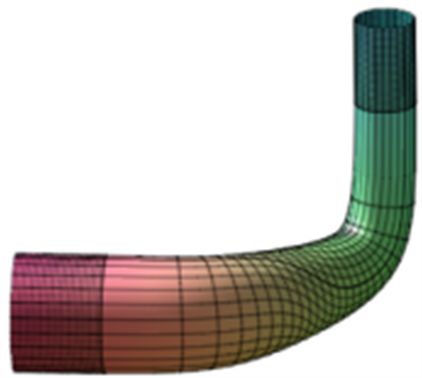Figure 1. Blending graph G0 with rational Bézier curve as axis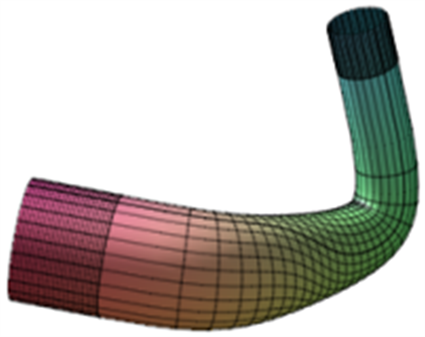Figure 2. Blending graph G1 with rational Bézier curve as axis

4. 结束语

Rational Bézier Curve and Its Application in Blending of Thickness Different Tubes[J]. 应用数学进展, 2018, 07(09): 1127-1132. https://doi.org/10.12677/AAM.2018.79130

1. 1. Warren, J. (1989) Blending Algebraic Surfaces. ACM Transactions on Graphics, 8, 263-278. https://doi.org/10.1145/77269.77270

2. 2. 吴文俊, 王定康. CAGD中的代数曲面拟合问题[J]. 数学的实践与认识, 1994(3): 26-31.

3. 3. Wallner, J. and Pottmann, H. (1997) Rational Blending Surfaces between Quadrics. CAGD, 14, 407-419. https://doi.org/10.1016/S0167-8396(96)00037-4

4. 4. Wu, T.R. and Zhou, Y.S. (2000) On Blending of Several Quadratic Algebraic Surfaces. CAGD, 17, 759-766. https://doi.org/10.1016/S0167-8396(00)00023-6

5. 5. Bai, G.-Z., Wang, H. and Yin, Z.-J. (2014) Employing Generalized Bezier Tube to Smoothly Blending Tubes Whose Axes Are Non-coplanar. Applied Mechanics and Materials, 513-517, 2301-2306. https://doi.org/10.4028/www.scientific.net/AMM.513-517.2301

6. 6. Wang, H. and Bai, G.-Z. (2013) Employing Generalized Cylindrical Helicoid Tube to Smoothly Blending Tubes Whose Axes Are Non-Coplanar. Applied Mechanics and Materials, 380-384, 1750-1754. https://doi.org/10.4028/www.scientific.net/AMM.380-384.1750

7. 7. Bai, G.-Z., Liu, S.-Y., Wang, H., Zhi, W. and Yin, Z.-J. (2014) A Novel Method for Smooth Blending Cylindrical Surfaces Whose Axes Are Non-Coplanar Based on Smooth Blending Axes. Applied Mechanics and Materials, 687-691, 1470-1473. https://doi.org/10.4028/www.scientific.net/AMM.687-691.1470

8. 8. Bai, G.-Z., Wang, H., Wu, Z., Yin, Z.-J. and Liu, S.-Y. (2014) Smoothly Blending of Two Elliptic Cylinders Whose Axes Are Non-Coplanar. Applied Mechanics and Materials, 644-650, 309-312. https://doi.org/10.4028/www.scientific.net/AMM.644-650.309

9. 9. Bai, G.Z., Wu, Z. and Lin, X. (2017) Intersecting Line of Conical Surface and Smoothly Blending of Two Tubes Whose Axes Are Non-Coplanar. Journal of Applied Mathematics and Physics, 5, 1887-1891. https://doi.org/10.4236/jamp.2017.59158

10. 10. 王芳, 白根柱. 有理Bézier曲线及其应用[J]. 应用数学进展, 2017, 6(8): 935-941.

11. NOTES

*通讯作者。